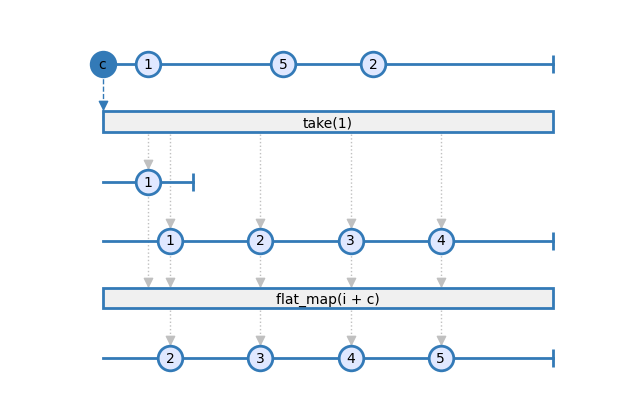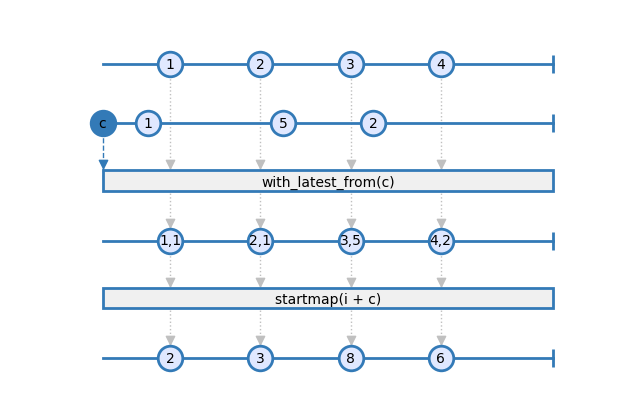# How To¶

This section contains examples of common operations in RxSci and Maki Nage. You can find the associated code for most of them here.

## Import and Export Data¶

### Read a csv file¶

You can work directly on CSV files (vs using Kafka as a source and sink), via the load_from_csv factory operator.

First import the csv module that contains all csv operators:

```import rxsci.container.csv as csv
```

Then you must declare the schema of the CSV file. Below is the schema of the iris dataset that you can retrieve from kaggle.

```iris_parser = csv.create_line_parser(
dtype=[
('id', 'int'),
('sepal_length_cm', 'float'),
('sepal_width_cm', 'float'),
('petal_length_cm', 'float'),
('petal_width_cm', 'float'),
('species', 'str'),
]
)
```

The create_line_parser operator supports options to customize the parser, such as the text encoding, column separator, and default values. See the documentation for more information.

```iris_data = csv.load_from_file('./Iris.csv', iris_parser)
```

### Write a csv file¶

The dump_to_file operator writes each input item to a row of a CSV file. The input items must be namedtuples. So first ensure that your data is structured as a namedtuple:

```IrisFeature = namedtuple('IrisFeature', ['id', 'species', 'sepal_ratio', 'petal_ratio'])

iris_features = iris_data.pipe(
rs.ops.map(lambda i: IrisFeature(
id=i.id, species=i.species,
sepal_ratio=i.sepal_length_cm / i.sepal_width_cm,
petal_ratio=i.petal_length_cm / i.petal_width_cm
)),
)
```

The dump_to_file operator uses the fields of the namedtuple to infer the columns names of the CSV file:

```iris_features.pipe(
csv.dump_to_file('iris_features.csv', encoding='utf-8'),
).subscribe()
```

## Extend Maki Nage¶

### Create an operator by composition¶

The simplest way to create a new operator is by composing other existing operators. Let’s consider these three operations done on some text input:

```rs.ops.map(lambda i: i.replace("-", " "))
rs.ops.filter(lambda i: 'bill' not in i)
rs.ops.map(lambda i: i.capitalize())
```

The natural way to use them is by chaining them in a pipe:

```data.pipe(
rs.ops.map(lambda i: i.replace("-", " "))
rs.ops.filter(lambda i: 'bill' not in i)
rs.ops.map(lambda i: i.capitalize())
)
```

But as more and more transforms are added, you can end up with a very long pipe. You can easily improve the readability and reuse some operations by grouping operators. For example, the previous three operators can be grouped as a custom operator:

```def cleanup_text():
return rx.pipe(
rs.ops.map(lambda i: i.replace("-", " ")),
rs.ops.filter(lambda i: 'bill' not in i),
rs.ops.map(lambda i: i.capitalize()),
)
```

The function cleanup_text is an operator that you can use in a pipe:

```data = [
'hello',
'the-quick-brown-fox',
'bill is fast',
'lorem ipsum'
]

rx.from_(data).pipe(
cleanup_text()
).subscribe(on_next=print)
```
```Hello
The quick brown fox
Lorem ipsum
```

### Create a stateful operator by composition¶

Stateful operators are more complex to implement because they need to update a state. Hopefully, In many cases, you can create new operators by combining three base operators: scan, filter, and map:

• The scan operator updates the state.

• The filter operator controls when items must be emitted.

• The map operator emits the items from the state.

Let’s consider the following need: Sum all items up to some threshold. An item must be emitted each time the sum would cross the threshold. Then the sum process starts again:The state logic can be implemented with the following function:

```def _sum_split(acc, i):
if acc + i > threshold:
return (i, acc)
return (acc+i, None)
```

Here acc contains the state. It is a tuple where:

• The first field is the current sum

• The second field is the item to emit or None if nothing must be emitted.

The full implementation of the operator simply consists in combining scan, filter, and map in a wrapper function:

```def sum_split(threshold):
def _sum_split(acc, i):
if acc + i > threshold:
return (i, acc)
return (acc+i, None)

return rx.pipe(
rs.ops.scan(_sum_split, seed=(0, None)),
rs.ops.filter(lambda i: i is not None),
rs.ops.map(lambda i: i),
)
```

You can now use sum_split just as any builtin operator:

```data = [1, 2, 3, 4, 1, 2, 6, 1]

rx.from_(data).pipe(
sum_and_split(5)
).subscribe(print)
```
```3 3 5 2 6
```

## Use the configuration parameters¶

The operators of a Maki Nage application have access to the configuration settings. The configuration is passed as the first argument of the operator. It is an Observable that emits configuration objects. These objects are dicts and they correspond to the content of the YAML configuration file. If the configuration file is read locally, then only one item is emitted. However if the configuration file is served from consul, then one item is emitted each time a change is made in consul.

So the application can choose to use the initial configuration for its whole life, or to dynamically adapt to changes in the configuration.

### Use only the initial configuration¶

Using only the initial configuration is the simplest way use the configuration settings. This way of working means that the application must be restarted to take into account changes in the configuration file.

The following code shows such an implementation:

```def my_operator(config, data):
initial_config = config.pipe(
ops.take(1),
)

result = stage2_config.pipe(
ops.flat_map(lambda c: data.pipe(
# my operations, whith c variable available in this scope
rs.ops.map(lambda i: i + c['config']['increment_value'])
)
)

return result,
```

In this example, the initial_config observable is first created. This observable emits only the first configuration item. Then the computation graph is started from this configuration item, and wrapped in a flat_map scope. Within the scope of flat_map, all operators have access to the c variable. This variable is the dict corresponding to the configuration file.

See this marble diagram for a visual explanation each step:### Use the configuration dynamically¶

Since the configuration argument is an Observable, it is possible to dynamically adjust the application behavior without having to restart it.

This mode of operation requires that the source items are combined with the configuration items. Then operations can be done on these tuples of item/configuration. Here is the same example that on the previous section, but with the lastest configuration being always used to do the computation:

```def my_operator(config, data):
result = data.pipe(
rs.ops.with_latest_from(config),
rs.ops.starmap(lambda i, c: i + c['config']['increment_value']),
)

return result,
```

See this marble diagram for a visual explanation each step: